# Analysis of a light beam refracted by a glass plate

in StemSocial4 months ago (edited)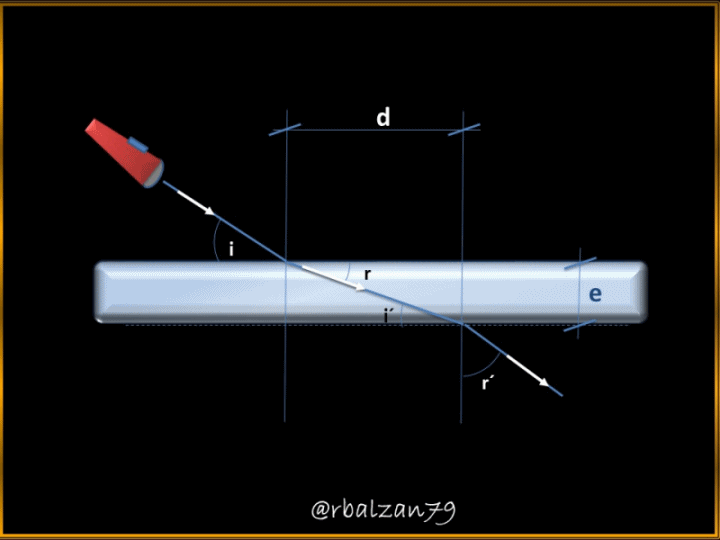Greetings my dear friends I hope you are all well, as we have already expressed in other opportunities, there are many essential phenomena for the existence of humanity, among them, the light, this wonderful phenomenon nothing more and nothing less, is responsible for transporting the images to our retinas for the optic nerve to transfer it to our brain and we can observe our environment in all its splendor, this expressed in a general way.

We can say then, that through the propagation of any ray of light, other intrinsic phenomena are developed at the same time, due to the presence of any type of obstacles that may absorb, reflect, refract, among other actions to the light that transports the images to our ocular system, remember that the phenomenon of light belongs to the important family of electromagnetic radiation, which propagate in the form of waves and also manage to propagate in the vacuum.

Exercise

A person carefully observes how a ray of light strikes a certain sheet of glass, whose thickness is 15 cm, also observing that the ray of light strikes the sheet at an angle of 45 °, considering that the material or elastic medium that surrounds the glass sheet is the air, this glass sheet has a refractive index of 1.45, therefore, in relation to the above, answer the following questions:

a.- What would be the angle at which the light beam will exit from such a glass sheet?

b.- What will be the exit distance of the light beam relative to the point of incidence of the beam?

Solución

Data:
i = 45 ° (Angle of incidence).
n = 1,45 (Refractive index of the glass sheet).
e = 15 cm (Glass sheet thickness).
Light beam exit angle = ?
Distance between the point of incidence and the exit point of the light beam = ?

Before starting to answer these questions, it is important that we can make a small figure or image that allows us to better visualize the approach of our statement: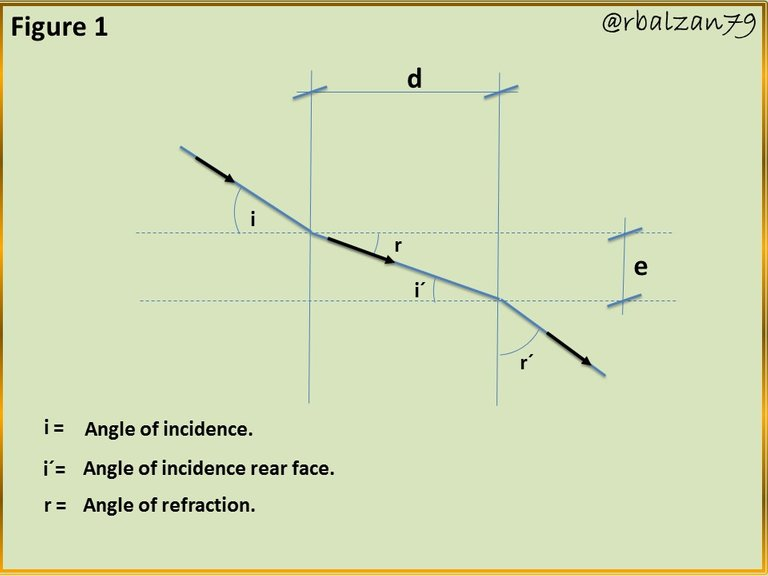a.- To answer the first question we must follow the path of the light ray as it propagates through the two material media described above (air and glass) and for this, we will link with the law of refraction (Snell's Law), so let's know its formulation: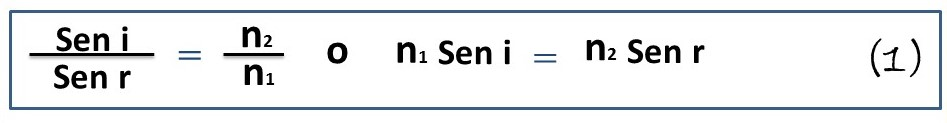Highlighting that n1 and n2, represent the refractive indices of the material or elastic media related to such propagation (air and glass sheet), thus: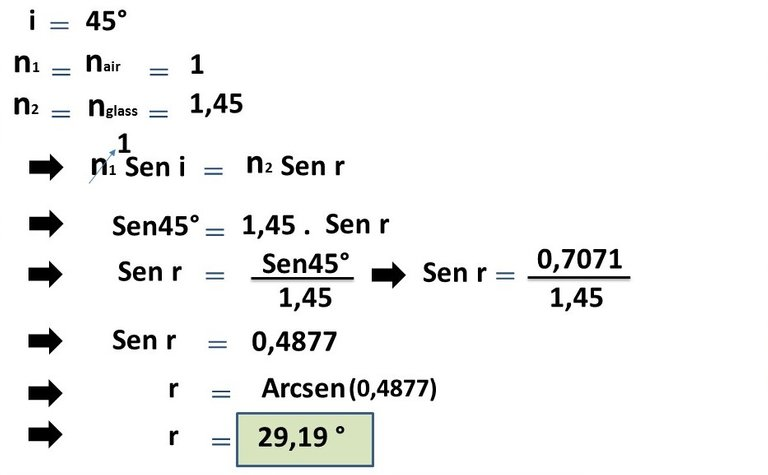In this way we find our first angle indicated as refraction angle (r), to continue knowing the other angles, if we look closely at our figure 1, we can see how the angle at which the light beam strikes the second side of the glass sheet is equal to the angle found above, ie, r = 29, 19 °, thus:Now we have to find the angle at which the light beam exits the glass sheet indicated above and, for this purpose, we apply formula 1 again, but now with the following relation: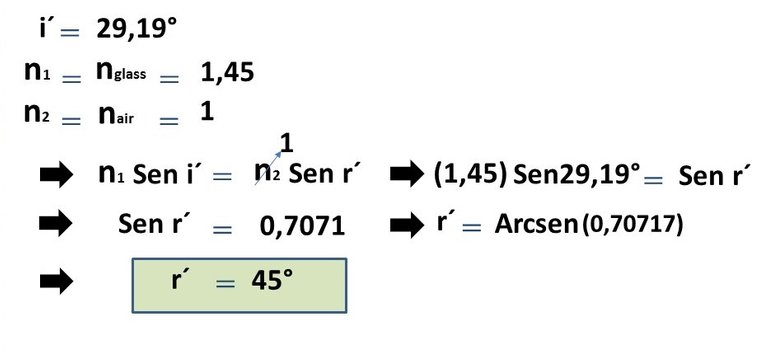Now we have calculated the requested angle, that is, the angle at which the light ray exited the glass plate represented by (r') = 45°, therefore, it has the same value of the angle that the light ray experienced when it hit the glass plate (i = 45°), this with respect to the normal to the glass plate and, as you could see in Figure 1 above.

b.- For our second question, it is necessary that we can observe again our schematic figure (Figure 1), and in it, we can notice how the trajectory followed by the light ray inside the sheet corresponds to the hypotenuse of a right triangle, where, one of those legs corresponds to our question, therefore, we have the following relation:Then we clear our variable of interest (d):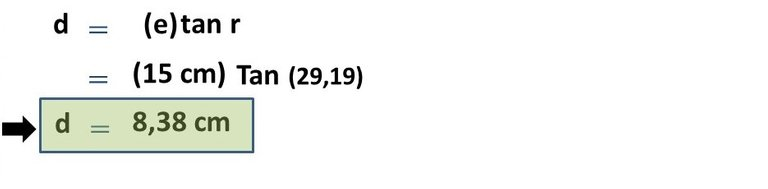In this way we were able to locate the distance between the point of incidence and the exit point of the light beam passing through the light sheet, which is 8.38 cm, therefore, we noticed a refraction of the light beam.

# Conclusion

Any ray of light, when propagating can experience some type of intrinsic phenomenon to such propagation, among them, refraction as we could notice or observe on this occasion, and for this, we used the law of refraction or Snell's law, this allowed us to follow the route or path of propagation of the ray and knowing the respective angles on each of the faces of the sheet indicated and thus the distance between the point of incidence of the light beam and the exit point of the light beam.

To locate the value of the distance from the exit point of the beam, we would have used the value of the angle (i'), as it is similar to the angle (r), as previously explained, and also because they are two right triangles divided or sharing the same hypotenuse, hypotenuse that in this case served us to obtain the required distance, that is, 8.38 cm exist between the point of incidence and exit point of the light beam analyzed.

Until another opportunity my dear friends.

Note: The images were created by the author @rbalzan79 using Power Point and Paint and the animated gif using PhotoScape.

# Recommended bibliographic references

 Light - Reflection and Refraction. Link.